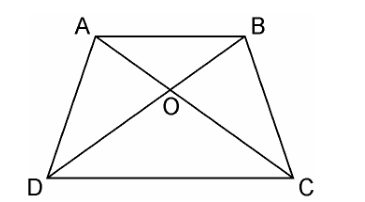Guru

# Diagonals AC and BD of a trapezium ABCD with AB || DC intersect each other at the point O. Using a similarity criterion for two triangles, show that AO/OC = OB/OD Q.3

• 0

For class 10 Triangles chapter of exercise 6.3 How i solve this question in easy way because i don’t know how to solve this problem Diagonals AC and BD of a trapezium ABCD with AB || DC intersect each other at the point O. Using a similarity criterion for two triangles, show that AO/OC = OB/OD

Share

1.In ΔDOC and ΔBOA,

AB || CD, thus alternate interior angles will be equal,

∴∠CDO = ∠ABO

Similarly,

∠DCO = ∠BAO

Also, for the two triangles ΔDOC and ΔBOA, vertically opposite angles will be equal;

∴∠DOC = ∠BOA

Hence, by AAA similarity criterion,

ΔDOC ~ ΔBOA

Thus, the corresponding sides are proportional.

DO/BO = OC/OA

⇒OA/OC = OB/OD

Hence, proved.

• 0
2.In ΔDOC and ΔBOA,

AB || CD, thus alternate interior angles will be equal,

∴∠CDO = ∠ABO

Similarly,

∠DCO = ∠BAO

Also, for the two triangles ΔDOC and ΔBOA, vertically opposite angles will be equal;

∴∠DOC = ∠BOA

Hence, by AAA similarity criterion,

ΔDOC ~ ΔBOA

Thus, the corresponding sides are proportional.

DO/BO = OC/OA

⇒OA/OC = OB/OD

Hence, proved.

• 0